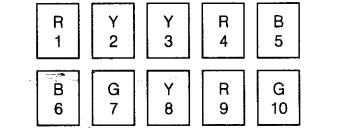# Sonia picks up a card from

Question:

Sonia picks up a card from the given cardsFind the probability of getting

(a) an odd number

(b) a Y card

(c) a G card

(d) a B card bearing number greater than 7.

Solution:

(a) The probability of getting an odd number

$=\frac{\text { Number of events getting an odd number }}{\text { Total number of events }}=\frac{5}{10}=\frac{1}{2}$

(b) The probability of getting a $Y$ card

$=\frac{\text { Number of events getting a } Y \text { card }}{\text { Total number of events }}=\frac{3}{10}$

(c) The probability of getting a Gcard

$=\frac{\text { Number of events getting a G card }}{\text { Total number of events }}=\frac{2}{10}=\frac{1}{5}$

(d) The probability of getting a B card bearing number greater than 7

$=\frac{\text { Number of events getting a } B \text { card bearing number greater than } 7}{\text { Total number of events }}=\frac{0}{10}=0$

Identify which symbol should appear in each sector of questions 113 and 114.